##### Ti-84 Plus Graphing Calculator For Dummies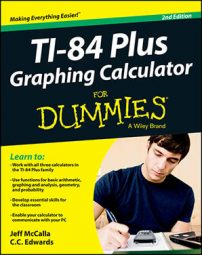Press [MATH] to access the Math MATH submenu on your TI-84 Plus calculator. This submenu contains general mathematical functions you can insert into an expression.

## Converting between decimals and fractions on the TI-84 Plus

The Frac function always converts a finite decimal to a fraction. If your calculator can’t convert a decimal to a fraction, it lets you know by redisplaying the decimal. Be sure to enter the decimal before inserting the Frac function. Press [MATH][ENTER][ENTER] to quickly convert a decimal answer to a fraction as shown in the first screen.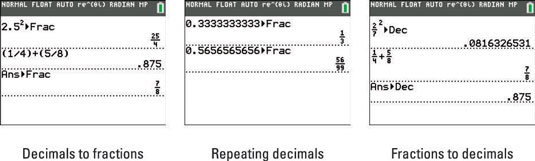How do you convert an infinite repeating decimal into a fraction? Just type at least ten digits of the repeating decimal and press [MATH][ENTER][ENTER]. See the second screen.

The Dec function converts a fraction to a decimal. Enter the fraction before you insert the Dec function. See the third screen.

If you are in Automatic mode, include a decimal in an expression to produce a decimal answer.

## Cubing and taking cube roots on the TI-84 Plus

The cube function cubes the value that precedes the function. The cube function is rarely used because it is easier to press [^] to cube an expression. The cube-root template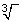finds the cube root of a value that follows the function. See the first screen.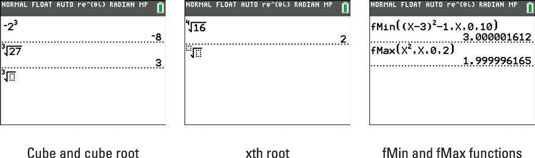## Taking the xth root on the TI-84 Plus calculator

The xth root template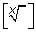finds the xth root of the value that follows the function. To use this function, first enter the root x, then insert the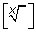function, and then enter the argument. Alternatively, on the TI-84 Plus C you can insert the xth root template first. Then type the root, press the right-arrow key, and type the expression. This is illustrated in the second screen.

## Finding the location of maximum and minimum values on the TI-84 Plus

The fMin and fMax functions approximate where the minimum or maximum value of a function occurs in a specified interval. They do not compute the minimum or maximum value of the function; they just give you the x-coordinate of the minimum or maximum point.

The fMin and fMax functions are stand-alone functions in the sense that they cannot be used in an expression. To use these functions, insert the appropriate function, fMin or fMax, at the beginning of a new line on the Home screen. Then enter the definition of the function whose minimum or maximum you want to locate. Press [,] and enter the variable used in the definition of the function you just entered. Press [,] and enter the lower limit of the specified interval. Then press [,], enter the upper limit, and press [)].

Finally, press [ENTER] to approximate the location of the minimum or maximum in the specified interval. This is illustrated in the third screen. In this screen the calculator is approximating the location of the maximum value of the function x2 in the interval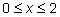## Using numerical differentiation and integration templates on the TI-84 Plus

The calculator cannot perform symbolic differentiation and integration. For example, it can't tell you the derivative of x2 is 2x, nor can it evaluate an indefinite integral. But the nDeriv template will approximate the derivative (slope) of a function at a specified value of the variable, and the fnInt template will approximate a definite integral.

Insert the nDeriv template. Templates are intuitive to use. First, enter the variable you want to take the derivative with respect to and then press the right-arrow key. Enter the function whose derivative you want to find and then press the right-arrow key. Then enter the value at which the derivative is to be taken. Finally, press [ENTER] to approximate the derivative. This is illustrated in the first screen.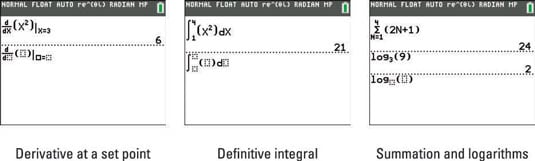To use the fnInt template, insert fnInt. Enter the lower limit and press the right-arrow key, then enter the upper limit and press the right-arrow key. Enter the function you’re integrating and press the right-arrow key. Enter the variable used in the definition of the function you just entered. Finally, press [ENTER] to approximate the definite integral. This is illustrated in the second screen.

The calculator may give you an error message or a false answer if nDeriv is used to find the derivative at a nondifferentiable point or if fnInt is used to evaluate an improper integral.

## Using the TI-84 Plus calculator's summation and logarithm templates

These templates can be found by pressing the up-arrow key to scroll in the MATH menu, or by pressing [ALPHA][WINDOW] to access the templates in the shortcut menu.

The summation template can be used to find the sum of a sequence. In math classrooms, this is commonly known as Sigma notation. The template should look exactly like a Sigma notation problem in your math textbook.

To use the summation template, insert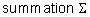Notice the cursor has a blinking “A” indicating your calculator is in Alpha mode. Press the key that corresponds to the variable you want to use and press the right-arrow key. Enter the lower limit, press the right-arrow key, then enter the upper limit and press the right-arrow key again. Enter the expression and press [ENTER] to find the sum of the sequence as shown in the first line of the last screen.

Using the logarithm template, you can change the base of a logarithm! Press the following keys to insert the logBASE template: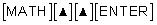Simply enter the base, press the right-arrow key, and enter the number you wish to take the logarithm of. Press [ENTER] to display the answer. Isn’t that easy and fun?